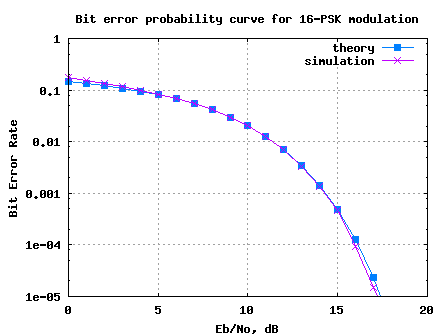- DSP log - http://www.dsplog.com -

Bit error rate for 16PSK modulation using Gray mapping

Posted By Krishna Sankar On May 18, 2008 @ 8:22 pm In Modulation | 40 Comments

In this post, let us derive the theoretical bit error probability for 16PSK modulation using Gray coded mapping. For deriving the equation, we will refer material from the following posts:

As discussed in the previous posts, the key feature of Gray code is that adjacent symbols differ by only one bit. The 16PSK constellation with Gray mapping can be as shown in the figure below.Figure: 16PSK constellation plot with Gray coded bit-mapping

From the discussion on symbol error rate for 16PSK in AWGN , we have proved that the symbol error rate for an M-PSK modulation is,

.

## Relation between bit energy Eb/No to symbol energy Es/No

As can be seen from the constellation plot, for a 16PSK modulation, each symbol transmits bits. In general, for an M-PSK modulation the number bits in each constellation symbol is,

.

Since each symbol consists of bits, the symbol to noise ratio is times the bit to noise ratio , ie.

.

## Relation between symbol error and bit error

For reasonable symbol to noise ratio , the symbol will be in error when noise causes the symbol to fall in the adjacent symbol bin. Now thanks to the Gray coded mapping, even if the symbol goes into the adjacent symbol there will be only 1 bit in error from the bits. So, the relation between symbol error and bit error is,

.

Note:

For very low symbol to noise ratio, the noise can cause the symbol to fall into the non-adjacent symbol bin. This may cause more than 1 bit error for each symbol error. However, we can ignore this case considering that we are interested only in reasonably high values of symbol to noise ratio.## Probability of bit error for M-PSK modulation

Combining the above two equations, the bit error probability for a general M-PSK modulation is,

.

(Of course for 16-PSK, M=16).

## Matlab/Octave script for simulation 16-PSK BER

The Matlab/Octave script performs the following:

(a) Generation of random 1′s and 0′s

(b) Assigning group of 4 bits to each constellation symbol per the Gray mapping

(c) Addition of white Gaussian Noise

(d) Demodulation of PSK symbols and

(e) De-mapping per decimal to Gray conversion

(f) Counting the number of bit errors

(g) Running this for each value of Eb/No in steps of 1dB.Figure: Bit error rate plot for 16PSK modulation using Gray mapping

Observations

1. The simulated results show good agreement with the theoretical results.

2. For low Eb/No values, we can see that the simulated bit error rate is slightly higher than that expected by theory. As explained above, this can be attributed to noise causing symbol to fall into the non-adjacent bin.

Hope this helps,
Krishna

URL to article: http://www.dsplog.com/2008/05/18/bit-error-rate-for-16psk-modulation-using-gray-mapping/

URLs in this post:

 Symbol Error Rate for 16PSK: http://www.dsplog.com/2008/03/18/symbol-error-rate-for-16psk/

 Binary to Gray code conversion for PSK: http://www.dsplog.com/2008/05/11/binary-to-gray-code-conversion-psk-pam/

 Matlab/Octave script for simulating 16PSK bit error rate: http://www.dsplog.com/db-install/wp-content/uploads/2008/05/script_16psk_gray_mapping_bit_error_rate.m